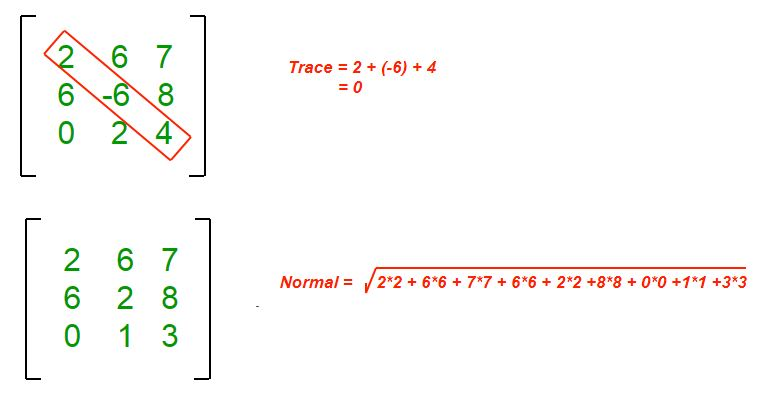# Java Program to Find the Normal and Trace of a Matrix

For a given 2D matrix, the purpose is to find the Trace and Normal of the matrix.

Normal of a matrix is defined as the square root of the sum of squares of all the elements of the matrix.

Trace of a given square matrix is defined as the sum of all the elements in the diagonal.

Examples :

```Input : matrix[][] = {{1, 4, 4},
{2, 3, 7},
{0, 5, 1}};
Output : Normal = 11
Trace  = 5
Explanation :
Normal = sqrt(1*1+ 4*4 + 4*4 + 2*2 +
3*3 + 7*7 + 0*0 + 5*5 + 1*1)
= 11
Trace  = 1+3+1 = 5

Input :matrix[][] = {{8, 9, 11},
{0, 1, 15},
{4, 10, -7}};
Output : Normal = 25
Trace  = 2
Explanation :
Normal = sqrt(8*8 +9*9 + 11*11 + 0*0 + 1*1 +
15*15 + 4*4 + 10*10 + -7*-7) = 25
Trace  = (8+1-7) = 2```Example:

## Java

 `// Java program to find the trace` `// and normal of the given matrix`   `import` `java.io.*;`   `class` `geeksforgeeks {`   `    ``// Dimension of the given matrix` `    ``static` `int` `max = ``50``;`   `    ``// Finds Normal of the given` `    ``// matrix of size N x N` `    ``static` `int` `Normal(``int` `matrix[][], ``int` `N)` `    ``{` `        ``// Initializing sum` `        ``int` `s = ``0``;` `        ``for` `(``int` `j = ``0``; j < N; j++)` `            ``for` `(``int` `k = ``0``; k < N; k++)` `                ``s += matrix[j][k] * matrix[j][k];` `        ``return` `(``int``)Math.sqrt(s);` `    ``}`   `    ``// Finds trace of the given` `    ``// matrix of size N x N` `    ``static` `int` `Trace(``int` `matrix[][], ``int` `N)` `    ``{` `        ``int` `s = ``0``;` `        ``for` `(``int` `j = ``0``; j < N; j++)` `            ``s += matrix[j][j];` `        ``return` `s;` `    ``}`   `    ``// The Driver code` `    ``public` `static` `void` `main(String[] args)` `    ``{`   `        ``int` `matrix[][] = {` `            ``{ ``2``, ``3``, ``5``, ``6``, ``7` `},      { ``8``, ``9``, ``10``, ``11``, ``12` `},` `            ``{ ``13``, ``14``, ``15``, ``16``, ``17` `}, { ``18``, ``1``, ``3``, ``0``, ``6` `},` `            ``{ ``7``, ``8``, ``11``, ``8``, ``11` `},` `        ``};`   `        ``System.out.println(``"Trace of the Matrix is: "` `                           ``+ Trace(matrix, ``5``));` `        ``System.out.println(``"Normal of the Matrix is: "` `                           ``+ Normal(matrix, ``5``));` `    ``}` `}`

Output

```Trace of the Matrix is: 37
Normal of the Matrix is: 50```

Time Complexity: O(N*N), as we are using nested loops for traversing the matrix.

Auxiliary Space: O(1), as we are not using any extra space.

Feeling lost in the world of random DSA topics, wasting time without progress? It's time for a change! Join our DSA course, where we'll guide you on an exciting journey to master DSA efficiently and on schedule.
Ready to dive in? Explore our Free Demo Content and join our DSA course, trusted by over 100,000 geeks!

Previous
Next